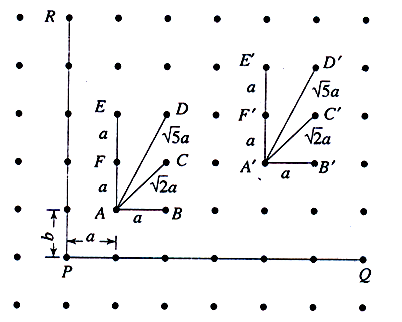## Pages

### WHAT IS CRYSTAL STRUCTURE?

Matter exists in three states viz. solids, liquids and  gases.  All these states are composed of atoms and molecules.  In case of solids, they are classified into many types based on several properties like  electrical, mechanical, magnetic, optical, thermal  etc.  The main reason for these different properties of solids is their crystal structure.

Crystal Structure
Periodic arrangement of atoms/ions over large atomic distances. Leads to structure displaying  LONG-RANGE ORDER that is Measurable and Quantifiable.

CRYSTAL

It is a substance in which the constituent particles are arranged in a systematic geometrical pattern.

SPACE LATTICE OR CRYSTAL LATTICE

A lattice is a regular and periodic arrangement of points in three dimension.  It is defined as an infinite array of points in three dimension in which every point has surroundings identical to that of every other point in the array.  The Space lattice is otherwise called the Crystal lattice.Consider the points P, Q and R.
Let us join the points P and Q by a straight line, and the point P and R by another straight line.
The line PQ is taken as X-axis and the line PR is taken as Y-axis.
The distance between any two successive lattice points in the X-direction is taken as `a’.

Similarly, the distance between any two successive lattice points along the Y-direction is taken as `b’.
Here a and b are said to be lattice translational vectors. Consider a square lattice in which a = b.

Consider two sets of points A, B, C, D, E, F and A', B', C', D', E', F'. In these two sets, the surrounding environment looks symmetrical; i.e. the distances AB and A'B', AC and A'C', AD and A'D', AE and A'E' and AF and A'F' are equal.

#### 1 comment:

1.